×#### Thank you for registering.

One of our academic counsellors will contact you within 1 working day.

Click to Chat

1800-1023-196

+91-120-4616500

CART 0

• 0

MY CART (5)

Use Coupon: CART20 and get 20% off on all online Study Material

ITEM
DETAILS
MRP
DISCOUNT
FINAL PRICE
Total Price: Rs.

There are no items in this cart.
Continue Shopping• Complete JEE Main/Advanced Course and Test Series
• OFFERED PRICE: Rs. 15,900
• View Details

```Revision Notes on Whole Numbers

Natural Numbers

All the positive counting numbers starting from one are called Natural Numbers.Predecessor and Successor

If we add 1 to any natural number, we get the next number, which is called the Successor of that number.

12 + 1 = 13

So 13 is the successor of 12.

If we subtract 1 from any natural number, we get the predecessor of that number.

12 – 1 = 11

So 11 is the predecessor of 12.

Remark: There is no predecessor of 1 in natural numbers.

Whole Numbers

Whole numbers are the collection of natural numbers including zero. So, the zero is the predecessor of 1 in the whole numbers.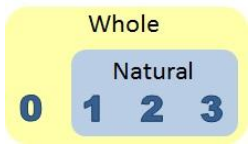Number Line

To draw a number line, follow these steps-

Draw a line and mark a point 0 on it.

Now mark the second point to the right of zero and label it as 1.

The distance between the 0 and 1 is called the unit distance.

Now you can mark other points as 2, 3, 4 and so on with the unit distance.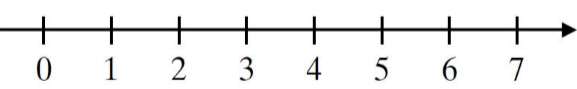This is the number line for the whole numbers.

1. The distance between two points

The distance between 3 and 5 is 2 units. Likewise, the distance between 1 and 6 is 5 units.

2. The greater number on the number line

The number on the right is always greater than the number on the left.

As number 5 is on the right of the number 2, Hence 5>2.

3. A smaller number on the number line

The number on the left of any number is always smaller than that number.

As number 3 is on the left of 7, so 3 < 7.

If we have to add 2 and 5, then start with 2 and make 5 jumps to the right. As our 5th jump is at 7 so the answer is 7.The sum of 2 and 5 is 2 + 5 = 7

Subtraction on the Number Line

If we have to subtract 6 from 10, then we have to start from 10 and make 6 jumps to the left. As our 6th jump is at 4, so the answer is 4.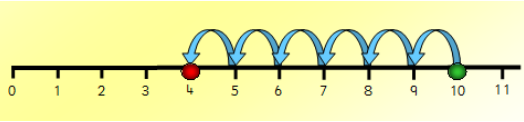The subtraction of 6 from 10 is 10 – 6 = 4.

Multiplication on the Number Line

If we have to multiply 4 and 3, then Start from 0, make 4 jumps using 3 units at a time to the right, as you reach to 12. So, we say, 3 × 4 = 12.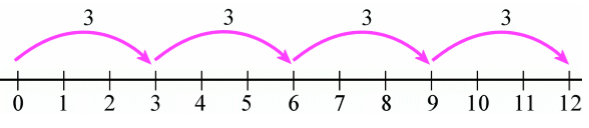Properties of Whole Numbers

1. Closure Property

Two whole numbers are said to be closed if their operation is also the whole number.

Operation
Meaning
Example
Closed or not

Whole numbers are closed under addition as their sum is also a whole number.

2 + 5 = 7

Yes

Subtraction

Whole numbers are not closed under subtraction as their difference is not always a whole number.

9 – 2 = 7

2 – 9 = (-7) which is not a whole number.

No

Multiplication

Whole numbers are closed under multiplication as their product is also a whole number.

9 × 5 = 45

Yes

Division

Whole numbers are not closed under division as their result is not always a whole number.

5 ÷ 1 = 5

5 ÷ 2 =, not a whole number.

No

2. Commutative Property

Two whole numbers are said to be commutative if their result remains the same even if we swap the positions of the numbers.

Operation
Meaning
Example
Commutative  or not

The addition is commutative for whole numbers as their sum remains the same even if we interchange the position of the numbers.

2 + 5 = 7

5 + 2 = 7

Yes

Subtraction

Subtraction is not commutative for whole numbers as their difference may be different if we interchange the position of the numbers.

9 – 2 = 7

2 – 9 = (-7) which is not a whole number.

No

Multiplication

Multiplication is commutative for whole numbers as their product remains the same even if we interchange the position of the numbers.

9 × 5 = 45

5 × 9 = 45

Yes

Division

The division is not commutative for whole numbers as their result may be different if we interchange the position of the numbers.

5 ÷ 1 = 5

1 ÷ 5 =, not a whole number.

No

3. Associative Property

The two whole numbers are said to be associative if the result remains the same even if we change the grouping of the numbers.

Operation
Meaning
Example
Associative  or not

The addition is associative for whole numbers as their sum remains the same even if we change the grouping of the numbers.

3 + (2 + 5) = (3 + 2) + 5

3 + 7 = 5 + 5

10 = 10

Yes

subtraction

Subtraction is not associative for whole numbers as their difference may change if we change the grouping of the numbers.

8 – (10 – 2) ≠ (8 – 10) – 2

8 - (8) ≠ (-2) – 2

0 ≠ (-4)

No

Multiplication

Multiplication is associative for whole numbers as their product remains the same even if we change the grouping of the numbers.

3 × (5 × 2) = (3 × 5) × 2

3 × (10) = (15) × 2

30 = 30

Yes

Division

The division is not associative for whole numbers as their result may change if we change the grouping of the numbers.

24 ÷ 3 ≠ 4 ÷ 2

8 ≠ 2

No

4. Distributivity of Multiplication over Addition

This property says that if we have three whole numbers x, y and z, then

x(y + z) = xy + xz

Example

Evaluate 15 × 45

Solution

15 × 45 = 15 × (40 + 5)

= 15 × 40 + 15 × 5

= 600 + 75

= 675

If we add zero to any whole number the result will the same number only. So zero is the additive identity of whole numbers.

a + 0 = 0 + a = a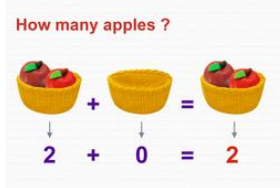This clearly shows that if we add zero apples to 2 apples we get the two apples only.

6. Identity for Multiplication

If we multiply one to any whole number the result will be the same whole number. So one is the multiplicative identity of whole numbers.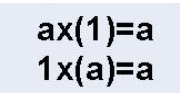Patterns

Patterns are used for easy verbal calculations and to understand the numbers better.

We can arrange the numbers using dots in elementary shapes like triangle, square, rectangle and line.

1. We can arrange every number using dots in a line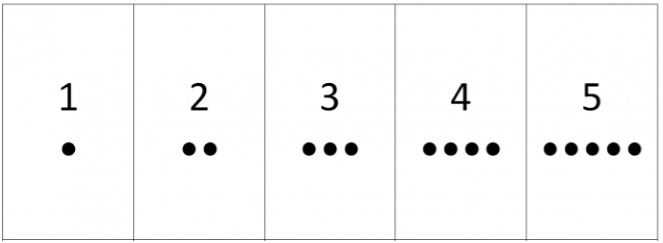2. We can arrange some numbers using a rectangle.3. We can arrange some numbers using a square.4. We can arrange some numbers using a triangle.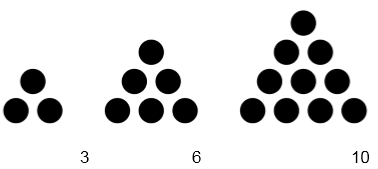Use of Patterns

Patterns can be used to simplify the process.

1. 123 + 9 = 123 + 10 - 1 = 133 -1 = 132

123 + 99 = 123 + 100 – 1 = 223 – 1 = 222

2. 83 × 9 = 83 × (10-1) = 830 – 83 = 747

83 × 99 = 83 × (100-1) = 8300 – 83 = 8217
```### Course Features

• 728 Video Lectures
• Revision Notes
• Previous Year Papers
• Mind Map
• Study Planner
• NCERT Solutions
• Discussion Forum
• Test paper with Video Solution